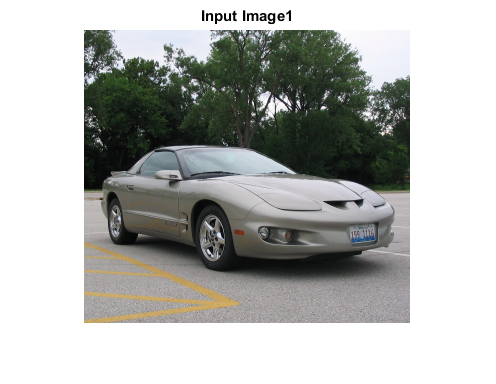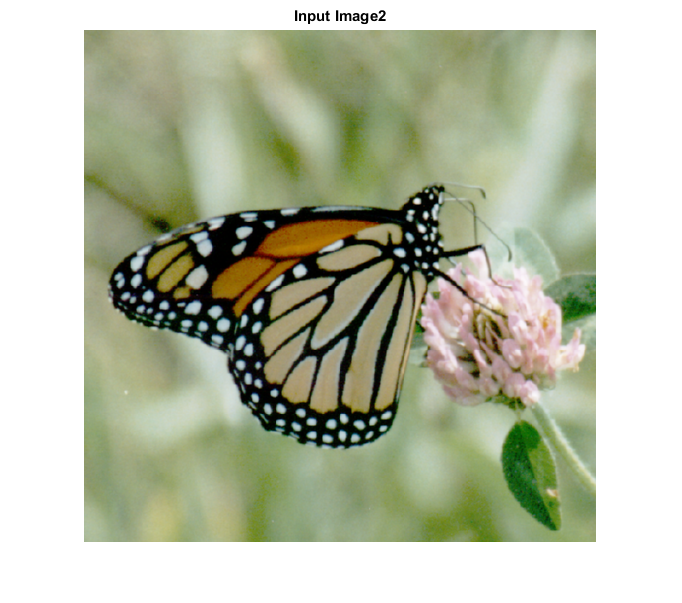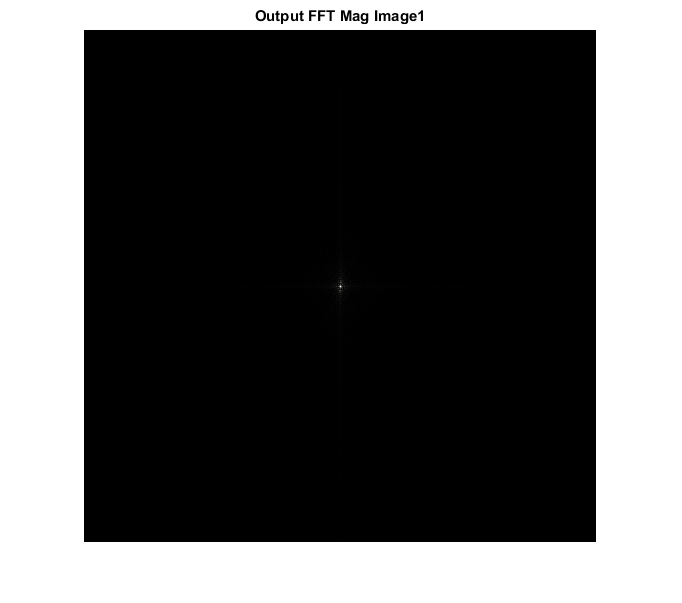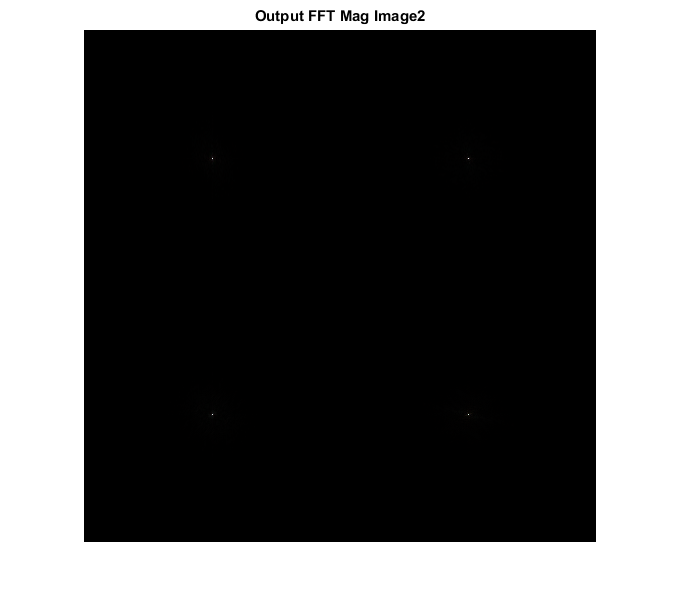# FFT_MAG_CVIP

fft_mag_cvip() - extract magnitude of the Fourier spectrum.

## SYNTAX

`mag = fft_mag_cvip( input_img, block_size )`

Input Parameters include:

• input_img - The orignial image which can be grayscale or RGB.
• block_size - Should be a power of 2 or []. if empty, then functions finds the nearest power of 2 and zero pad the image, to that block size. Any other value smaller than image size will partiotion the image to windows of that block size and FFT will be calculated separately in each window.

Output Parameter include:

• mag - Magnitude of FFT.

## DESCRIPTION

The function performs an FFT on the input image of a block size specified by the user and returns only the magnitude information of the resultant spectrum.

## REFERENCE

1. Scott E Umbaugh. DIGITAL IMAGE PROCESSING AND ANALYSIS: Applications with MATLAB and CVIPtools, 3rd Edition.

## EXAMPLE

```% Read image

% Block size

block_size1 = [];

block_size2 = 256;

% Call function

spect1 = fft_mag_cvip( input_img1, block_size1 );

spect2 = fft_mag_cvip( input_img2, block_size2 );

S1 = remap_cvip(spect1);

S2 = remap_cvip(spect2);

% Display input image

figure; imshow(input_img1);title('Input Image1');

figure;imshow(input_img2);title('Input Image2');

% Display output image

figure; imshow(S1,[]);title('Output FFT Mag Image1');

figure;imshow(S2,[]);title('Output FFT Mag Image2');
```